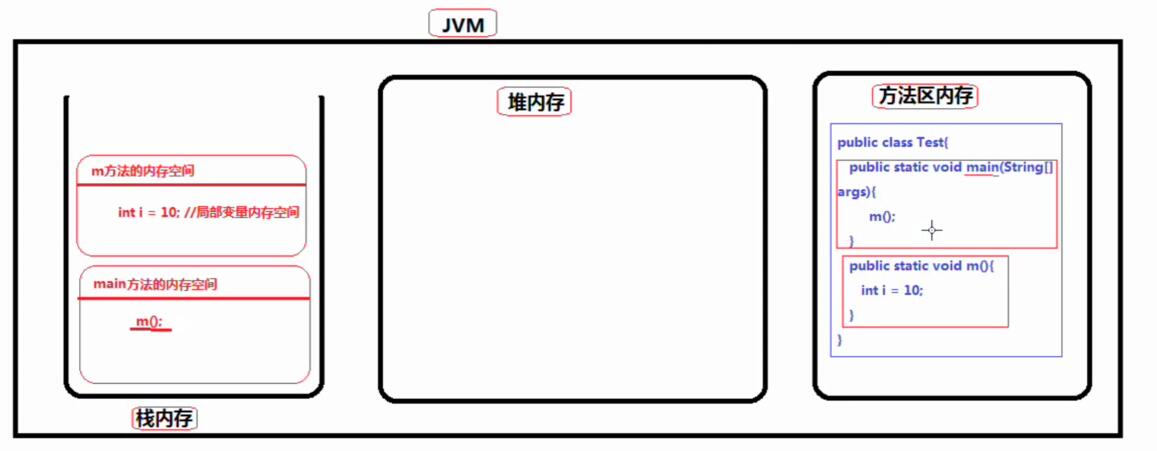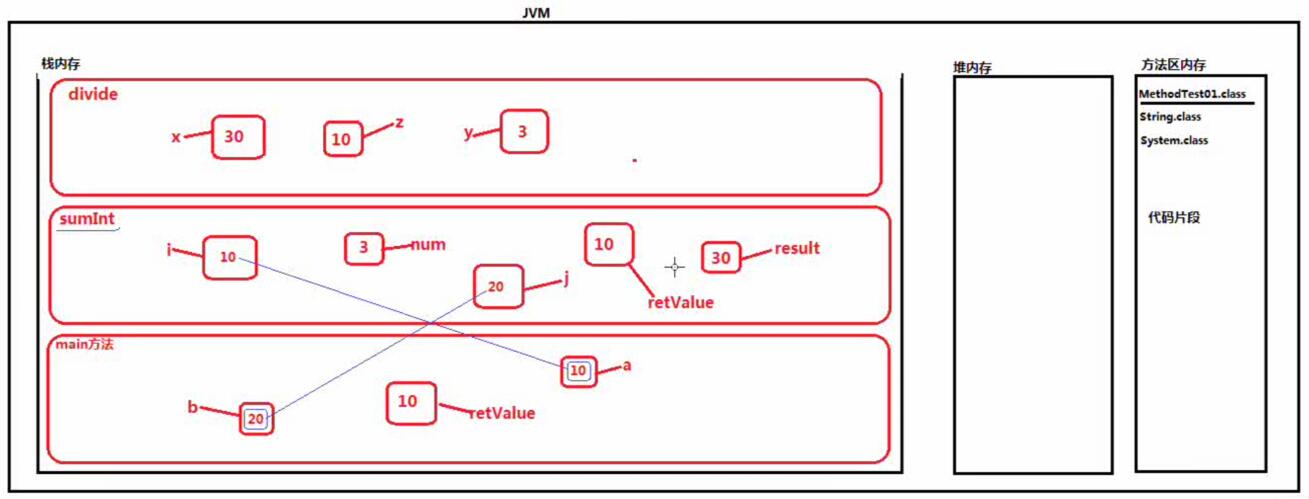（1）在MyEclipse中字体是红色的是一个类的名字，并且这个类除了我们自定义的类是JavaSE类库中自带的

（2）其实JavaSE类库中自带的类，例如：String.class\System.class,这些类的类名也是标识符

（3）只要是类名就一定是标识符

public class D29_{

public static void main(String[] args) {

int a = 10;

int b = 20;

int retValue = sunInt(a,b);

System.out.println(retValue);

}

public static int sumInt(int i ,int j) {

int result = i + j;

int num = 3;

int retValue = divide(result,num);

return retValue;

}

public static int divide(int x,int y) {

int z = x/y;

return z;

}

}public class D29_{

​

public static void main(String[] args) {

int result1 = sumInt2(1,2);

System.out.println(result1);

double result2 = sumDouble(1.0,2.0);

System.out.println(result2);

long result3 = sumLong(1L,2L);

System.out.println(result3);

}

public static int sumInt2(int a, int b) {

return a+b;

}

public static double sumDouble(double a,double b) {

return a+b;

}

public static long sumLong(long a ,long b) {

return a+b;

}

｝2.改写代码，具体解析下次更新再说。

public class D29_{

public static void main(String[] args) {

//调用方法的时候像在使用一个方法一样

//参数的类型不同，对应调用的方法不同

//此时区分方法不再依靠方法名依据的是参数的数据类型

System.out.println(sum(2,7));

System.out.println(sum(2.0,7.0));

System.out.println(sum(2L,7L));

}

public static int sum(int a,int b) {

return a+b;

}

public static double sum(double a ,double b) {

return a+b;

}

public static long sum(long a,long b) {

return a+b;

}

}